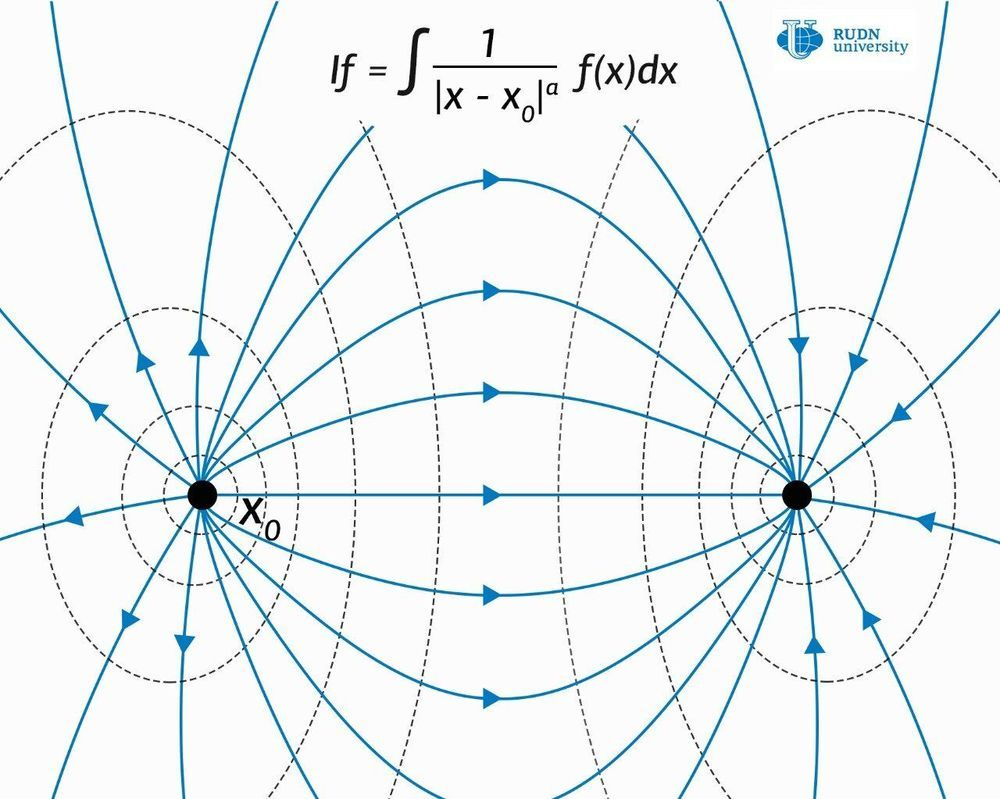Menu

## BlogRUDN University mathematicians have proven the Hardy-Littlewood-Sobolev (HLS) inequalities for the class of generalized Riesz potentials. These results extend the scope of these potentials in mathematics and physics because the main tools for working with such potentials are based on HLS inequalities. New mathematical tools can greatly simplify calculations in quantum mechanics and other fields of physics. The results of the study are published in the journal Mathematical Notes.

Modern physics describes the world in terms of fields and their potentials—that is, the values of the field at each point. But the physical quantities that we can measure are forces and accelerations, that is, derivatives of the second-order of the potential of the corresponding field. The problem of reconstructing the field configuration with the available values of forces and accelerations observed in experiments is complex and not always analytically solvable. Differentiation operations in multidimensional space—operators are usually used to describe the correlation between the potential of the field and the forces. In particular, electromagnetic and gravitational interactions are described in the language of operators.

Since the potential of the field can be determined up to a constant value, for the convenience of calculations, the initial value of the potential is taken at some point in multidimensional space, or on the border of any spatial area. But in some cases, mathematical models of such fields lead to a singularity, that is, at some points the value of the field becomes infinite, and therefore loses its physical meaning.